# DS Module 1.pptx

2 Jun 2023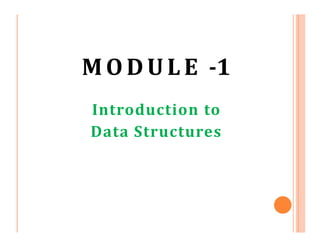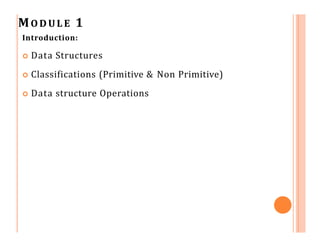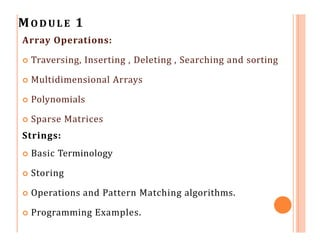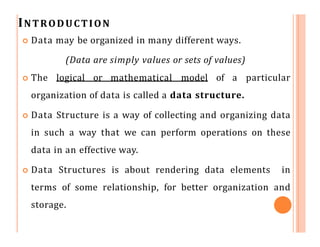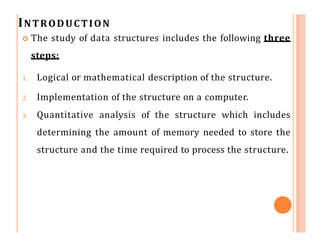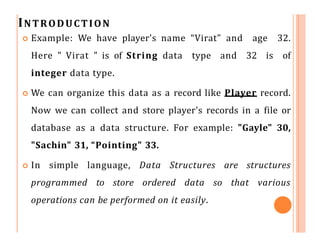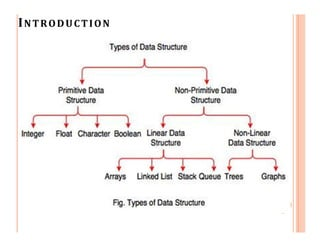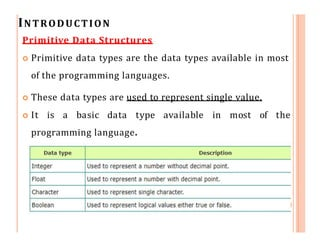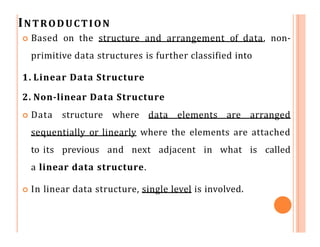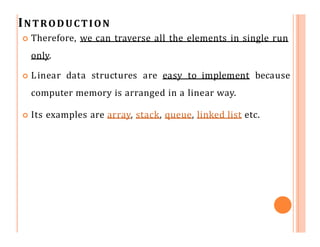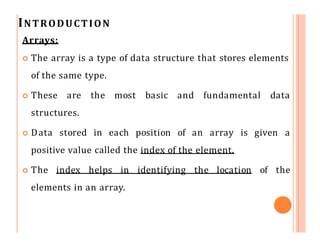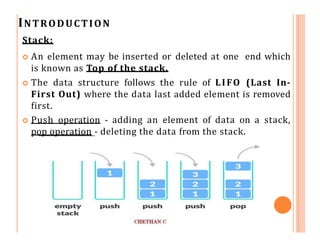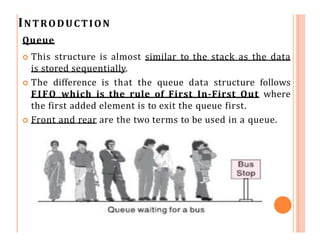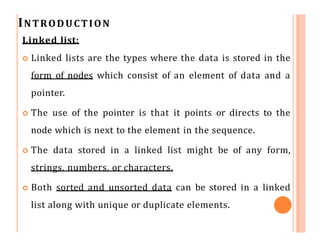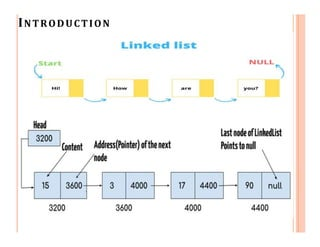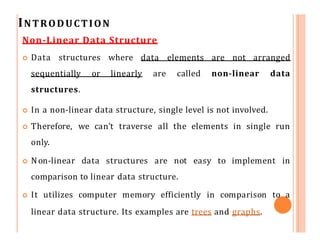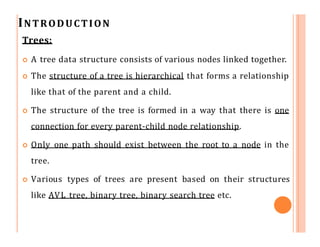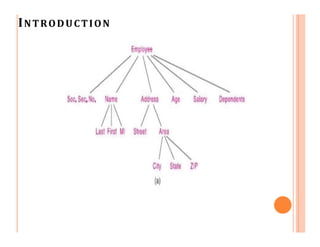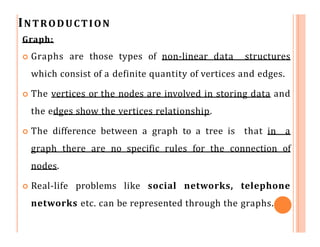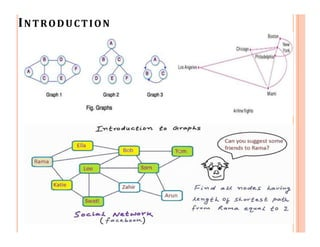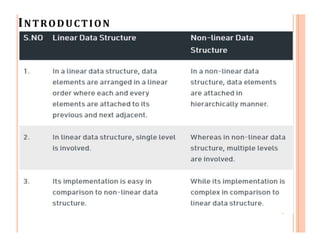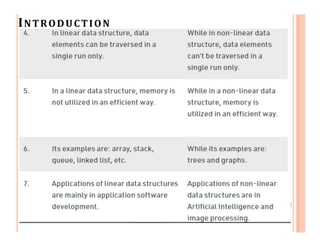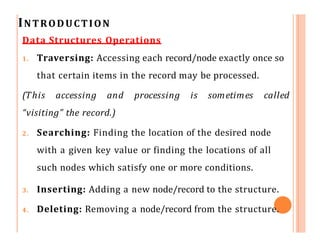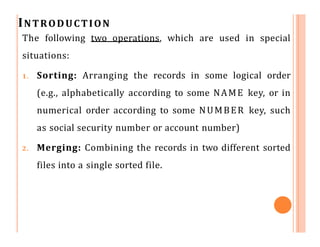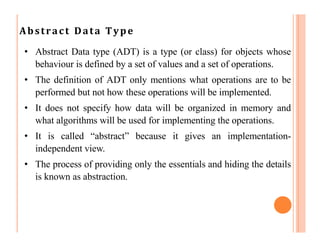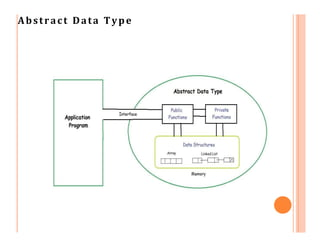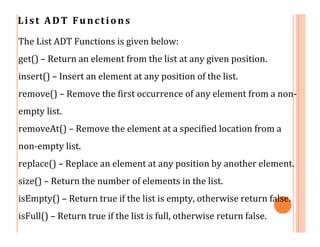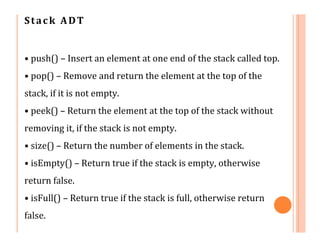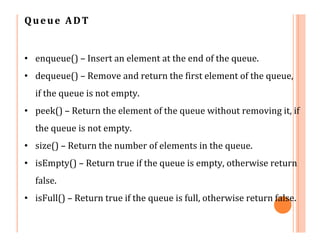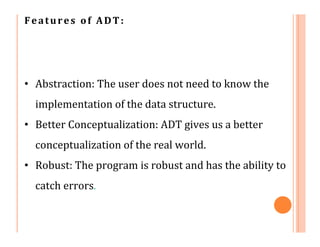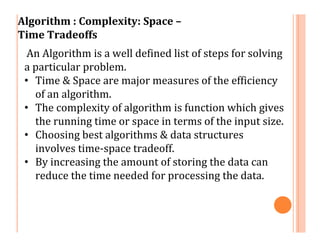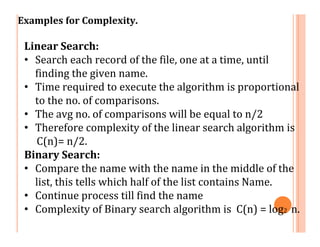1 sur 32

### DS Module 1.pptx

• 2. MO D U L E 1 Introduction:  Data Structures  Classifications (Primitive & Non Primitive)  Data structure Operations
• 3. MO D U L E 1 Array Operations:  Traversing, Inserting , Deleting , Searching and sorting  Multidimensional Arrays  Polynomials  Sparse Matrices Strings:  Basic Terminology  Storing  Operations and Pattern Matching algorithms.  Programming Examples.
• 4. INTRODUCTION  Data may be organized in many different ways. (Data are simply values or sets of values)  The logical or mathematical model of a particular organization of data is called a data structure.  Data Structure is a way of collecting and organizing data in such a way that we can perform operations on these data in an effective way.  Data Structures is about rendering data elements in terms of some relationship, for better organization and storage.
• 5. INTRODUCTION  The study of data structures includes the following three steps: 1. Logical or mathematical description of the structure. 2. Implementation of the structure on a computer. 3. Quantitative analysis of the structure which includes determining the amount of memory needed to store the structure and the time required to process the structure.
• 6. INTRODUCTION  Example: We have player's name “Virat" and age 32. Here " Virat " is of String data type and 32 is of integer data type.  We can organize this data as a record like Player record. Now we can collect and store player's records in a file or database as a data structure. For example: "Gayle" 30, "Sachin" 31, “Pointing" 33.  In simple language, Data Structures are structures programmed to store ordered data so that various operations can be performed on it easily.
• 8. INTRODUCTION Primitive Data Structures  Primitive data types are the data types available in most of the programming languages.  These data types are used to represent single value.  It is a basic data type available in most of the programming language.
• 9. INTRODUCTION  Based on the structure and arrangement of data, non- primitive data structures is further classified into 1. Linear Data Structure 2. Non-linear Data Structure  Data structure where data elements are arranged sequentially or linearly where the elements are attached to its previous and next adjacent in what is called a linear data structure.  In linear data structure, single level is involved.
• 10. INTRODUCTION  Therefore, we can traverse all the elements in single run only.  Linear data structures are easy to implement because computer memory is arranged in a linear way.  Its examples are array, stack, queue, linked list etc.
• 11. INTRODUCTION Arrays:  The array is a type of data structure that stores elements of the same type.  These are the most basic and fundamental data structures.  Data stored in each position of an array is given a positive value called the index of the element.  The index helps in identifying the location of the elements in an array.
• 12. INTRODUCTION Stack:  An element may be inserted or deleted at one end which is known as Top of the stack.  The data structure follows the rule of LIFO (Last In- First Out) where the data last added element is removed first.  Push operation - adding an element of data on a stack, pop operation - deleting the data from the stack.
• 13. INTRODUCTION Queue  This structure is almost similar to the stack as the data is stored sequentially.  The difference is that the queue data structure follows FIFO which is the rule of First In-First Out where the first added element is to exit the queue first.  Front and rear are the two terms to be used in a queue.
• 14. INTRODUCTION Linked list:  Linked lists are the types where the data is stored in the form of nodes which consist of an element of data and a pointer.  The use of the pointer is that it points or directs to the node which is next to the element in the sequence.  The data stored in a linked list might be of any form, strings, numbers, or characters.  Both sorted and unsorted data can be stored in a linked list along with unique or duplicate elements.
• 16. INTRODUCTION Non-Linear Data Structure  Data structures where data elements are not arranged sequentially or linearly are called non-linear data structures.  In a non-linear data structure, single level is not involved.  Therefore, we can’t traverse all the elements in single run only.  Non-linear data structures are not easy to implement in comparison to linear data structure.  It utilizes computer memory efficiently in comparison to a linear data structure. Its examples are trees and graphs.
• 17. INTRODUCTION Trees:  A tree data structure consists of various nodes linked together.  The structure of a tree is hierarchical that forms a relationship like that of the parent and a child.  The structure of the tree is formed in a way that there is one connection for every parent-child node relationship.  Only one path should exist between the root to a node in the tree.  Various types of trees are present based on their structures like AVL tree, binary tree, binary search tree etc.
• 19. INTRODUCTION Graph:  Graphs are those types of non-linear data structures which consist of a definite quantity of vertices and edges.  The vertices or the nodes are involved in storing data and the edges show the vertices relationship.  The difference between a graph to a tree is that in a graph there are no specific rules for the connection of nodes.  Real-life problems like social networks, telephone networks etc. can be represented through the graphs.
• 23. INTRODUCTION Data Structures Operations 1. Traversing: Accessing each record/node exactly once so that certain items in the record may be processed. (This accessing and processing is sometimes called “visiting” the record.) 2. Searching: Finding the location of the desired node with a given key value or finding the locations of all such nodes which satisfy one or more conditions. 3. Inserting: Adding a new node/record to the structure. 4. Deleting: Removing a node/record from the structure.
• 24. INTRODUCTION The following two operations, which are used in special situations: 1. Sorting: Arranging the records in some logical order (e.g., alphabetically according to some NAME key, or in numerical order according to some NUMBER key, such as social security number or account number) 2. Merging: Combining the records in two different sorted files into a single sorted file.
• 25. Abstract Data Type • Abstract Data type (ADT) is a type (or class) for objects whose behaviour is defined by a set of values and a set of operations. • The definition of ADT only mentions what operations are to be performed but not how these operations will be implemented. • It does not specify how data will be organized in memory and what algorithms will be used for implementing the operations. • It is called “abstract” because it gives an implementation- independent view. • The process of providing only the essentials and hiding the details is known as abstraction.
• 27. List ADT Functions The List ADT Functions is given below: get() – Return an element from the list at any given position. insert() – Insert an element at any position of the list. remove() – Remove the first occurrence of any element from a non- empty list. removeAt() – Remove the element at a specified location from a non-empty list. replace() – Replace an element at any position by another element. size() – Return the number of elements in the list. isEmpty() – Return true if the list is empty, otherwise return false. isFull() – Return true if the list is full, otherwise return false.
• 28. Stack ADT • push() – Insert an element at one end of the stack called top. • pop() – Remove and return the element at the top of the stack, if it is not empty. • peek() – Return the element at the top of the stack without removing it, if the stack is not empty. • size() – Return the number of elements in the stack. • isEmpty() – Return true if the stack is empty, otherwise return false. • isFull() – Return true if the stack is full, otherwise return false.
• 29. Queue ADT • enqueue() – Insert an element at the end of the queue. • dequeue() – Remove and return the first element of the queue, if the queue is not empty. • peek() – Return the element of the queue without removing it, if the queue is not empty. • size() – Return the number of elements in the queue. • isEmpty() – Return true if the queue is empty, otherwise return false. • isFull() – Return true if the queue is full, otherwise return false.
• 30. Features of ADT: • Abstraction: The user does not need to know the implementation of the data structure. • Better Conceptualization: ADT gives us a better conceptualization of the real world. • Robust: The program is robust and has the ability to catch errors.
• 31. Algorithm : Complexity: Space – Time Tradeoffs An Algorithm is a well defined list of steps for solving a particular problem. • Time & Space are major measures of the efficiency of an algorithm. • The complexity of algorithm is function which gives the running time or space in terms of the input size. • Choosing best algorithms & data structures involves time-space tradeoff. • By increasing the amount of storing the data can reduce the time needed for processing the data.
• 32. Examples for Complexity. Linear Search: • Search each record of the file, one at a time, until finding the given name. • Time required to execute the algorithm is proportional to the no. of comparisons. • The avg no. of comparisons will be equal to n/2 • Therefore complexity of the linear search algorithm is C(n)= n/2. Binary Search: • Compare the name with the name in the middle of the list, this tells which half of the list contains Name. • Continue process till find the name • Complexity of Binary search algorithm is C(n) = log2 n.